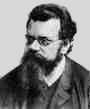Home

PHY 418: Statistical Mechanics I
Prof. S. Teitel stte@pas.rochester.edu ---- Spring 2012

## Problem Set 3

Due Wednesday, March 7, in lecture

• Problem 1 [10 points total]

In lecture we derived the canonical partition function QN(V,N) for the idea gas using a factorization method. One can also directly compute it by taking the Laplace transform of the microcanonical partition function Ω(E,V,N). Using our result from lecture,  Ω(E,V,N) = [ Vh3 (2πmE)3/2 ]N 1             [(3N/2) - 1]!  N! (Δ/E)

compute directly the Laplace transform,

 QN(T,V) = ∞∫0 dEΔ Ω(E,V,N)e-βE

and show that you get the same result as found in lecture.

• Problem 2 [20 points total]

In lecture we discussed the canonical ensemble, in which the temperature T, volume V, and number of particle N of a system are fixed, while the energy E is allowed to fluctuate. Suppose now that you wish to describe a system in which the temperature T, number of particles N, and pressure p are fixed, while the volume V is allowed to fluctuate. This would describe a system in contact with a thermal and mechanical reservior, in which the wall separating the system and the reservior is heat conducting and moveable. We can call this case the constant pressure ensemble.

a) Define the appropriate partition function Z(T, p, N) of the system in this new constant pressure ensemble. [5 points]

b) If you defined Z properly in part (a), then the Gibbs free energy should be given by

G(T, p, N) = -kBT ln Z(T, p, N)

To demonstrate this, show that using G defined from Z as above, the average volume of the system is correctly given by,

<V> = (∂G/∂p)T,N      [5 points]

c) Derive a relation, in this constant pressure ensemble, between the isothermal compressibility κT and fluctuations in the volume V of the system. Show from this relation that the relative fluctuation in V vanishes in the thermodynamic limit. [5 points]

d) Consider an ideal gas of non-relativistic, non-interacting, point particles of mass m. Explicitly compute the partition function Z(T,p,N) of this gas. Use Z to compute G(T,p,N), and then from G compute the specific heat at constant pressure, Cp. Show that you get the correct answer for the ideal gas. [5 points]

• Problem 3 [20 points total]

Consider a classical gas of N indistinguishable non-interacting particles with ultrarelativistic energies, i.e. their kinetic energy - momentum relation is given by ε = pc, with c the speed of light and p the magnitude of the particle's momentum. The gas is confined to a box of volume V.

(a) Compute the canonical partition function for this system. [5 points]

(b) Show that this system obeys the usual ideal gas law, pV = NkBT. [5 points]

(c) Show that the total average energy is, E = 3NkBT (and hence using (b) gives, E/V = 3p). [5 points]

(d) Show that the ratio of specific heats is, Cp/CV = 4/3. [5 points]

• Problem 4 [20 points total]

Consider a three dimensional classical ideal gas of atoms of mass m, moving in a potential energy

 V(x) = { ½ αx2 +∞ x≥0    x<0

The infinite potential for x < 0 may be viewed as a rigid wall filling the y-z plane at x=0. The atoms, therefore, are attracted to this wall, but they move freely in the y and z directions. Let T be the temperature and n = N/A be the total number of atoms per unit area of the wall.

a) calculate the local atomic density n(x) (number of atoms per unit volume) as a function of the distance x from the wall. Note that

 n= ∞∫0 n(x) dx
[5 pts]

b) Show that the ideal gas law (relating pressure, temperature, and density) continues to hold locally everywhere. [5 pts]

c) Find the pressure that the atoms exert on the wall. How does this pressure vary with temperature? [5 pts]

d) Calculate the energy and specific heat per unit area of the wall. [5 pts]